## Simplifying transfer function...

Hi

I'm trying to rearrange a transfer function to get my s^2 term with other specific terms . Below is the denominator of the transfer function. I know that my goal is to get (Izz*u)/m for the s^2 term, so I divide by Df*Dr*(a+b)*u*m, as shown below.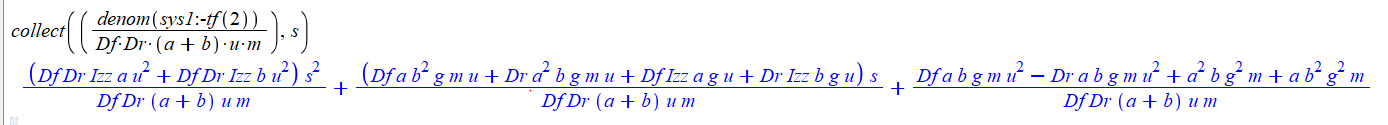However, if we look at the s^2 terms for now, the equation should be further simplified leaving me with simply (Izz*u)/m, but Maple doesn't want to do this for some reason, and if I use simplify on the above expression it tries to expand everything again, and forgetting about my collect(fun, s).

Is there any way to get around this?

Many thanks

## limit function different to limit series function...

Dear all

I have a function g that I compute its limit at x0

but when I compute series(g(x),x=x0) then I compute the limit of the series I get different limit

series_limit.mw

## How can I evaluate nested sum that includes piece...

Hi

I have a piecewise equation,and two Sum (one of them is infinity and the other has upperband k). I need a numerical value for F2.

H := r->; piecewise(r < 1, 1);

XF := x-> 2;

f1 := k-> sum(XF(j)*(1-H(j)), j = 0 .. k);

F2:=sum(f1(i), i = 0 .. infinity);

## Relabelling vertices of a graph sometimes doesn't ...

Hi,

This is a question more or less related to this one Is it possible to define variables with unusual na... but I think it's better to open a new thread. If some think otherwise, please feel free to displace it to the link above.

I want to relabel the vertices of a graph by using special characters.
It happens that this works perfectly if I do not impose the style of the drawing but that it doesn't if I set, for instance, spring=style.
In the attached file you will see that the subsitution of the old vertex names by the new ones doesn't work if apply it directly on the many operators of the PLOT command contains.

Is this behaviour fixed in more recent versions of Maple or it's still present?

Strange-Behaviour-with-SpringStyle.mw

## Texture fill. ...

Why does Maple not have texture fill for plotting. Even the old Pascal had it.

## Classic worksheet....

Maple Help points out

All Programs>Maple 2017>Classic Worksheet Maple 2017

link that I do not have. Is there a reason for this and how do I fix this?

## Error, reserved word `if` unexpected...

I don't understand whats wrong with my code
r(x):=proc(x)
local y
if (x=1)
then
y=1;
else
y=0;
end if;
return y
end proc

Error, reserved word `if` unexpected

I'm also having trouble getting this code to work:

h(x):=proc(x)
if (x= infinity)
then
return 1 ;
else
return 0;
end if;
end proc
theres no error but h(0) just returns h(0) not either value as I would like

## piecewise function in a loop...

Dear All,

How do I use the piecewise function z[k]:=piecewise(k<>0,0,k=0,1) in the loop

for k from 0 to 10 do

(sum((m^2-1)/z(z[k]+1),m,0..k))

end:

i want the value of z[k] to be automatically used in the loop for the corresponding value of k

## Graph the real roots...

Graph the real roots of the equation x3 + (a − 3)3x2 − a2x + a3=0 for a ∈ [0, 1].

Sol:=[solve(x^3+(a-3)^3*x^2-a^2*x+a^3=0,x)];

for i from 1 to 3 do

R||i:=unapply(Sol[i],a):

print(plot(R||i(a),a=0..1,numpoints=500)); end od:

Is there a simplier way to solve this. If not why did they choose this path?

## Ages Group JOHOR ......

 Ages Group JOHOR 0-4 118 5-9 174 10-14 374 15-19 597 20-24 670 25-29 677 30-34 516 35-39 407 40-44 291 45-49 196 50-54 155 55-59 101 60-64 66 65-69 34 70-74 21 >75 9 Grand Total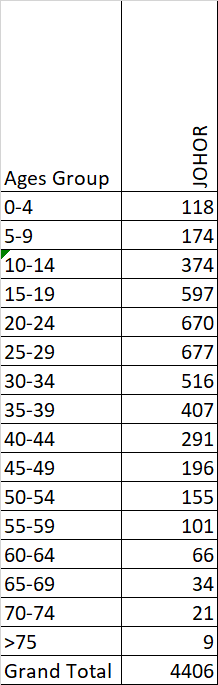Can you help me to make a 1D plotting by using this data?

## compute integral...

Dear all

Can we use maple to compute integral using the residual theorem

Compute_integral.mw

We can consider the contour a circle that contains one pole (Ipi/2)

Many thanks

## Clenshaw derivative recurrence...

Hi! I have been trying to calculate the value of theClenshaw derivative. I can get the regular clenshaw to work but not the derivative. I'm going off of the thread (https://scicomp.stackexchange.com/questions/27865/clenshaw-type-recurrence-for-derivative-of-chebyshev-series). (P.s notice I have halfed the A[z+1] term. I have tried both ways but the overall result is wrong so I am guessing something more important is wrong).

This is my code so far

```Clenshaw_Dx_1D:=proc(z,C,Nm,s)
local i,k,A,B: global Clen1D_Dx:

A := Vector(z..Nm+1+z);
B := Vector(z..Nm+1+z);

for k from Nm-1+z by -1 to 1+z do
A[k] := C[k] + 2*s*A[k+1] - A[k+2]:
od:

for k from Nm-1+z by -1 to 1+z do
B[k] := 2*A[k+1] + 2*s*B[k+1] - B[k+2]:
od:
Clen1D_Dx :=  A[z+1]/2 + s*B[1+z] - B[2+z]:

end:
```

Where z could be 0 or 1 depending on what index your C array starts at. C is the array of chebyshev coefficients of a Chebyshev series appromating u(x) for example. The Chebycoeff1D procedure calculates the Chebyshev coefficients  and the code below calls the procedure for a specific function. Try it with some values of xM, for example 4-10.

```Chebycoeff1D:=proc(express,Nn,C2,A,B)
local Cfac,fac1x,fac2x,k,K;

fac1x:=Pi/Nn;
for k from 1 to Nn do
Cfac(k):=eval(subs(x=evalf(cos(fac1x*(k-0.5)))*A+B,evalf(express)));
od; unassign('k'):
for K from 1 to Nn do
fac2x:=Pi*(K-1)/Nn;
od:
end:
```
```nn := 1.0:
Lc := 0.0: Rc := 1.0:
func:=nn*sin(2*Pi*x)+0.5*nn*sin(Pi*x):
Chebycoeff1D(func,xM+1,C,0.5*(Rc-Lc),0.5*(Rc+Lc)):
```

Once you have the C array call Clenshaw_Dx_1D. For example

`Clenshaw_Dx_1D(0,C,xM+1,0.0);  # Evalutes f'(x) in the middle of the domain.`

Check with

`subs(x=0.5, diff(func,x));`

The overall "pattern/shape" of the derivative values is correct, just not the amplitude/values. Any help here? Where is the Clenshaw derivative procedure going wrong.

## How do I plot complex transformations? ...

I have a region that can be graphed by A := plots:-inequal([evalc(abs(z)) < 3, evalc(1 < abs(z - 1))], x = -5 .. 5, y = -5 .. 5)

From there, I need to use the transformation

M := (3 + sqrt(5))/2*(z - x1)/(z - x2) where x1 := (9 - sqrt(45))/2 and x2 := (9 + sqrt(45))/2

How do I do this? Do I need to extract data points from the first graph? Is there an easier way to do this?

## How do I plot a hyperbola in polar with no asympto...

I would like to plot a hyperbola using the polarplot command, such as the following:

polarplot(3/(1-1.5*sin(theta)), coordinateview = [0 .. 10, 0 .. 2*Pi])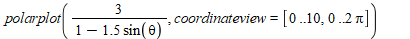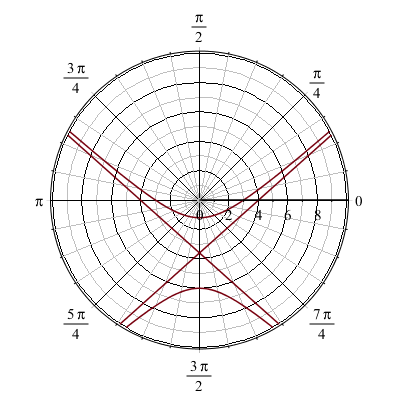But the graph includes the asymptotes, which I would not like to be included. I have tried the discont=true command, but it completely changes the shape of the graph and no longer looks like a hyperbola:

polarplot(3/(1-1.5*sin(theta)), coordinateview = [0 .. 10, 0 .. 2*Pi], discont = true)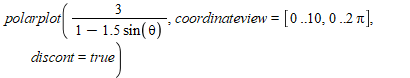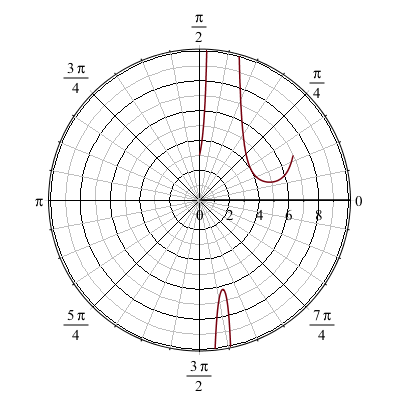How would I get the hyperbola above to display with no asymptotes?

Thanks